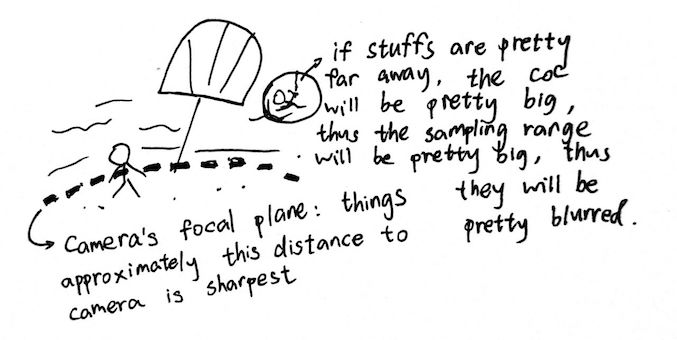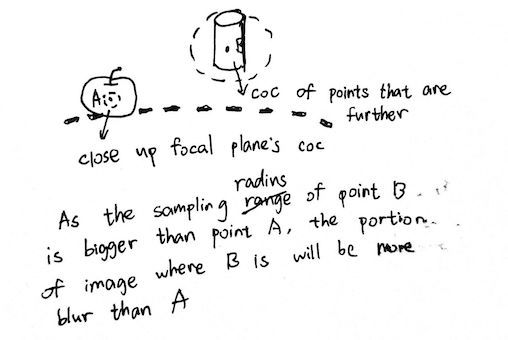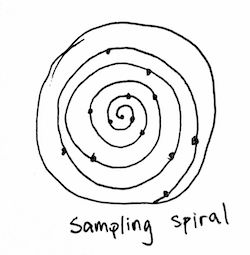A semi-curated blog of computer graphics and rendering.

This is a blog post from the past. Originally written at 2020-05-02.

Depth of field is usually used in cinematic scenes. Or when you are looking at things very, very small. It blurs the things the camera is not focusing. And I like things when they are small! And blurred! So this time, let’s take a look at this neat stuff, dof. First, let’s appreciate this masterpiece by P_Malin:## Introduction

DoF certainly adds a sense of depth, and it directs viewer’s attention onto certain stuffs. From what I’ve understand after reading P_Malin’s source code & his references documents, This is the gist about DoF:

1. The camera can only focus stuffs a certain distance away from the camera (named Plane in Focus)
2. If stuffs are outside of this region, the projection’s result will be a region instead of a point. The region is known as Circle of Confusion (CoC (not Clash of Clans))
3. Circle of Confusion increases as distance to Plane in Focus and/or aperture increases
4. As Circle of Confusion increases, our rays projects onto places further and further than the origin, making the image more blur.

That’s why we really need to calculate the Circle of Confusion before we march into any blurring steps. But first, it’s SCENE TIME!

float sol(vec3 pos) {
return pos.y;
}

float cube(vec3 pos, vec3 off) {
// finite repetition: https://www.iquilezles.org/www/articles/distfunctions/distfunctions.htm
vec3 c = vec3(3.0, 0.0, 3.0);
vec3 l = vec3(1.0);
pos = pos - c * clamp(floor(pos / c + 0.5), -l, l);
pos -= off;
vec3 d = abs(pos) - vec3(0.5);
return length(max(d, 0.0)) + min(max(d.x, max(d.y, d.z)), 0.0);
}

vec2 map(vec3 pos) {
float closest = 1000.0;
float id = -1.0;

float dist = sol(pos);
if (dist < closest) { closest = dist; id = 0.5; }

dist = cube(pos, vec3(0.0, 0.48, 0.0));
if (dist < closest) { closest = dist; id = 1.5; }

return vec2(closest, id);
}

vec2 trace(vec3 ro, vec3 rd) {
float depth = 0.0;
float id = -1.0;
for (int i = 0; i < 200; i++) {
vec2 info = map(ro + rd * depth);
if (abs(info.x) <= 0.001) {
id = info.y;
break;
}
depth += info.x;
}
return vec2(depth, id);
}

vec3 getFloorColor(vec3 pos) {
pos *= 2.0;
vec3 baseColor = vec3(1.0, 1.0, 1.0);
vec3 f = mod(floor(pos), 2.0);
return baseColor * clamp(abs(f.x - f.z), 0.8, 1.0);
}

vec3 getSkyColor(vec3 rd) {
vec3 baseColor = vec3(0.69, 0.89, 0.99);
return mix(baseColor, vec3(1.0, 1.0, 1.0), clamp(rd.y * 4.6, 0.0, 1.0));
}

vec3 getColor(float id, vec3 pos, vec3 rd) {
if (id < -0.5) { return getSkyColor(rd); } // sky
if (id < 1.0) { return getFloorColor(pos); } // ground
if (id < 2.0) { return vec3(1.0, 0.5, 0.0); }
return vec3(1.0, 0.0, 0.0); // red for undefined
}

vec3 getNormal(vec3 p) {
const float epsilon = 0.001;
float mapped = map(p).x;
return normalize(vec3(
mapped - map(vec3(p.x - epsilon, p.yz)).x,
mapped - map(vec3(p.x, p.y - epsilon, p.z)).x,
mapped - map(vec3(p.x, p.y, p.z - epsilon)).x
));
}

float getShadowIntensity(vec3 ro, vec3 rd) {
float depth = 0.001;
float res = 1.0;
for (int i = 0; i < 25; i++) {
float dist = map(ro + rd * depth).x;
res = min(res, 20.0 * dist / depth);
if (res < 1e-6) { break; }
depth += clamp(dist, 0.001, 2.0);
}
return clamp(res, 0.0, 1.0);
}

void main() {
vec2 xy = uv * 2.0 - 1.0;

vec3 ro = vec3(4.0 * sin(time), 1.5, 4.0 * cos(time));
vec3 center = vec3(0.0, 1.0, 0.0);

vec3 front = normalize(center - ro);
vec3 right = normalize(cross(front, vec3(0.0, 1.0, 0.0)));
vec3 up = normalize(cross(right, front));

mat4 lookAt = mat4(
vec4(right, 0.0),
vec4(up, 0.0),
vec4(front, 0.0),
vec4(0.0, 0.0, 0.0, 1.0)
);
vec3 rd = normalize(vec3(lookAt * vec4(xy, 2.0, 1.0)));

vec2 info = trace(ro, rd);
vec3 pos = ro + rd * info.x;
vec3 n = getNormal(pos);

vec3 lightDir = normalize(vec3(1.0, 1.0, 1.0));

float ambient = 1.0;
float diffuse = max(dot(n, lightDir), 0.0);
float dome = 0.2 + 0.8 * clamp(n.y, 0.0, 1.0);
float sol = 0.2 + 0.8 * clamp(-n.y, 0.0, 1.0);
float back = max(dot(n, vec3(-lightDir.x, 0.0, -lightDir.z)), 0.0);

vec3 light = vec3(1.0);
if (info.y > 0.0) {
light = vec3(0.0);
light += ambient * vec3(0.2, 0.2, 0.2) * shadow;
light += diffuse * vec3(0.82, 0.80, 0.82) * shadow;
light += dome * vec3(0.26, 0.32, 0.334);
light += sol * vec3(0.3, 0.21, 0.23);
light += back * vec3(0.2, 0.21, 0.23);
}

vec3 objColor = getColor(info.y, pos, rd) * light;
objColor = pow(objColor, vec3(0.4545));

color = vec4(objColor, 1.0);
}


Just a couple of cubes, nothing special. Let’s get started for real!

## Implementation

To first start the implementation, we should note that the most important thing we need to obtain is the Circle of Confusion (so that we can start sampling). According to this cool Meta CRT shader’s reference to GPU gems (which is a dead link there, BTW), here’s how we are going to calculate the circle of confusion:

$C = |A \frac{F(P - D)}{D(P - F)}|$

In which C stands for Circle of Confusion, A stands for Aperture/f-number, F stands for Focal Length, P stands for Focal Plane (which is the distance to camera we want to focus on), and D stands for Object Distance (which we already have - this is raymarching man!). Circle of Confusion itself acts like a blur radius, and it is affected by either distance to object or aperture/camera thingy.With all these stuffs, writing a circle of confusion calculation algorithm is easy:

float getCoC(float depth, float focalPlane) {
float focalLength = 0.02;
float aperture = min(1.0, focalPlane * focalPlane);
return abs(aperture * (focalLength * (focalPlane - depth)) /
(depth * (focalPlane - focalLength)));
}


Circle of Confusion itself acts kinda like a blurring radius. After obtaining the CoC value, all we need to do is randomly fetch texture colors around this position. Then, we can average it out (you can do it equally or by weight, it’s up to you):As I am looking at Paul’s code the whole time, let’s dissect his code here. After he obtains the CoC, he begins to sample points around it within the CoC radius. However, he does not do this randomly. He do this by forming a sampling spiral out from the center:What’s even cooler, is that he samples by using the golden ratio. This way, the sampling does not end up in a noticable pattern, and has no obvious bias. If you wanna know more about the golden ratio, you can check it out here. By sampling points like this inside the CoC circle, it satisfies our “the bigger the CoC circle is, the more blur it gets” requisite. So, that’s cool!

vec3 color = vec3(0.0);
float totalWeight = 0.0;
for (int i = 0; i < taps; i++) {
// radius is connected to index so it slowly becomes bigger and bigger
float radius = coc * sqrt(float(i)) / sqrt(float(taps));

// getting golden ratio position & adding it to current texcoord
float theta = float(i) * golden;
vec2 tapUV = v_texcoord + vec2(sin(theta), cos(theta)) * radius;

// sampling
vec4 tapped = texture2D(prevPass, tapUV);

// getting its depth
float tappedDepth = tapped.w;

// now contributing this part to color...
}


After sampling, now all we need to do is to calculate the color contribution of that particular texcoord to our final output color. We do this by calculating the weight of the texcoord, adding it to our final color output, and normalizing it by the total weight (so it doesn’t get way too bright or dark). But how do we get the weight? Well, there are multiple ways. But as P_Malin puts it, we can just use CoC as our weight! Why? Because we want the further places to “bloom” out further, right? And further places have a bigger CoC value! Thus there is no probs using CoC at all!

    ...
// getting its depth
float tappedDepth = tapped.w;

if (tappedDepth > 0.0) {
float tappedCoC = getCoC(tappedDepth, focalPlane);
float weight = max(0.001, tappedCoC);
color += tapped.rgb * weight;
totalWeight += weight;
}
}
color /= totalWeight;


Alas, the shader demo thing I wrote in my blog does not support (and I do not intend to) multiple passes, and the method I metioned above applies to two passes only. Or is it? Well no! Of course it supports single passes. We only need to know the final color and the depth of the given uv position. And how do we get to know the depth of a given texcoord? Well, we can always do this:

fragColor = vec4(color, depth);


So in two passes, this makes sense because well, we can just sample it. And in a single pass, we can also just return it as a vec4. However, this means the sampling process is going to be extremely laggy for single pass because there’s gonna be a hell load of raymarchings and lighting calculations, but it works! And there won’t even be any artifacts caused by texture! So, the next shader we have will have an EXTREMELY LAGGY WARNING:

float sol(vec3 pos) {
return pos.y;
}

float cube(vec3 pos, vec3 off) {
// finite repetition: https://www.iquilezles.org/www/articles/distfunctions/distfunctions.htm
vec3 c = vec3(3.0, 0.0, 3.0);
vec3 l = vec3(1.0);
pos = pos - c * clamp(floor(pos / c + 0.5), -l, l);
pos -= off;
vec3 d = abs(pos) - vec3(0.5);
return length(max(d, 0.0)) + min(max(d.x, max(d.y, d.z)), 0.0);
}

vec2 map(vec3 pos) {
float closest = 1000.0;
float id = -1.0;

float dist = sol(pos);
if (dist < closest) { closest = dist; id = 0.5; }

dist = cube(pos, vec3(0.0, 0.48, 0.0));
if (dist < closest) { closest = dist; id = 1.5; }

return vec2(closest, id);
}

vec2 trace(vec3 ro, vec3 rd) {
float depth = 0.0;
float id = -1.0;
for (int i = 0; i < 200; i++) {
vec2 info = map(ro + rd * depth);
if (abs(info.x) <= 0.001) {
id = info.y;
break;
}
depth += info.x;
}
return vec2(depth, id);
}

vec3 getFloorColor(vec3 pos) {
pos *= 2.0;
vec3 baseColor = vec3(1.0, 1.0, 1.0);
vec3 f = mod(floor(pos), 2.0);
return baseColor * clamp(abs(f.x - f.z), 0.8, 1.0);
}

vec3 getSkyColor(vec3 rd) {
vec3 baseColor = vec3(0.69, 0.89, 0.99);
return mix(baseColor, vec3(1.0, 1.0, 1.0), clamp(rd.y * 4.6, 0.0, 1.0));
}

vec3 getColor(float id, vec3 pos, vec3 rd) {
if (id < -0.5) { return getSkyColor(rd); } // sky
if (id < 1.0) { return getFloorColor(pos); } // ground
if (id < 2.0) { return vec3(1.0, 0.5, 0.0); }
return vec3(1.0, 0.0, 0.0); // red for undefined
}

vec3 getNormal(vec3 p) {
const float epsilon = 0.001;
float mapped = map(p).x;
return normalize(vec3(
mapped - map(vec3(p.x - epsilon, p.yz)).x,
mapped - map(vec3(p.x, p.y - epsilon, p.z)).x,
mapped - map(vec3(p.x, p.y, p.z - epsilon)).x
));
}

float getShadowIntensity(vec3 ro, vec3 rd) {
float depth = 0.001;
float res = 1.0;
for (int i = 0; i < 25; i++) {
float dist = map(ro + rd * depth).x;
res = min(res, 20.0 * dist / depth);
if (res < 1e-6) { break; }
depth += clamp(dist, 0.001, 2.0);
}
return clamp(res, 0.0, 1.0);
}

vec4 getFinalColor(vec2 uv) {
vec3 ro = vec3(4.0 * sin(time), 1.5, 4.0 * cos(time));
vec3 center = vec3(0.0, 1.0, 0.0);

vec3 front = normalize(center - ro);
vec3 right = normalize(cross(front, vec3(0.0, 1.0, 0.0)));
vec3 up = normalize(cross(right, front));

mat4 lookAt = mat4(
vec4(right, 0.0),
vec4(up, 0.0),
vec4(front, 0.0),
vec4(0.0, 0.0, 0.0, 1.0)
);
vec3 rd = normalize(vec3(lookAt * vec4(uv, 2.0, 1.0)));

vec2 info = trace(ro, rd);
vec3 pos = ro + rd * info.x;
vec3 n = getNormal(pos);

vec3 lightDir = normalize(vec3(1.0, 1.0, 1.0));

float ambient = 1.0;
float diffuse = max(dot(n, lightDir), 0.0);
float dome = 0.2 + 0.8 * clamp(n.y, 0.0, 1.0);
float sol = 0.2 + 0.8 * clamp(-n.y, 0.0, 1.0);
float back = max(dot(n, vec3(-lightDir.x, 0.0, -lightDir.z)), 0.0);

vec3 light = vec3(1.0);
if (info.y > 0.0) {
light = vec3(0.0);
light += ambient * vec3(0.2, 0.2, 0.2) * shadow;
light += diffuse * vec3(0.82, 0.80, 0.82) * shadow;
light += dome * vec3(0.26, 0.32, 0.334);
light += sol * vec3(0.3, 0.21, 0.23);
light += back * vec3(0.2, 0.21, 0.23);
}

vec3 objColor = getColor(info.y, pos, rd) * light;
objColor = pow(objColor, vec3(0.4545));
return vec4(objColor, info.x);
}

vec2 rand2d(vec2 uv) {
return fract(sin(vec2(
dot(uv, vec2(215.1616, 82.1225)),
dot(uv, vec2(12.345, 856.125))
)) * 41234.45) * 2.0 - 1.0;
}

float getCoC(float depth, float focalPlane) {
float focalLength = 0.1;
float aperture = min(1.0, focalPlane * focalPlane);
return abs(aperture * (focalLength * (focalPlane - depth)) /
(depth * (focalPlane - focalLength)));
}

void main() {
vec2 xy = uv * 2.0 - 1.0;

float depth = getFinalColor(xy).w;
float focalPlane = 3.9;
float coc = getCoC(depth, focalPlane);
const int taps = 32;
float golden = 3.141592 * (3.0 - sqrt(5.0));
vec3 outputColor = vec3(0.0);
float tot = 0.0;
for (int i = 0; i < taps; i++) {
float radius = coc * sqrt(float(i)) / sqrt(float(taps));
float theta = float(i) * golden;
vec2 tapUV = xy + vec2(sin(theta), cos(theta)) * radius;
vec4 tapped = getFinalColor(tapUV);
float tappedDepth = tapped.w;
if (tappedDepth > 0.0) {
float tappedCoC = getCoC(tappedDepth, focalPlane);
float weight = max(0.001, tappedCoC);
outputColor += tapped.rgb * weight;
tot += weight;
}
}
outputColor /= tot;
color = vec4(outputColor, 1.0);
}


## Conclusion

If you think the code here looks terrible, you can go to here and check out the code. It looks a little bit better (and cleaner).

To magnify the DoF effect, I use a focal length value of 0.1. Also the focal distance is a constant above; it’s just 3.9 so it approximately focuses on the center cube. If you want to make it camera like, you can always sample the center (not current frag coordinate, center) and get its depth and set it as the focal distance! Also you might want to change it slowly, so it really looks camera-like. Also, if your GPU can take it, bring the taps variable up to 64 samples above. It will look a little bit better! Well, that’s it!